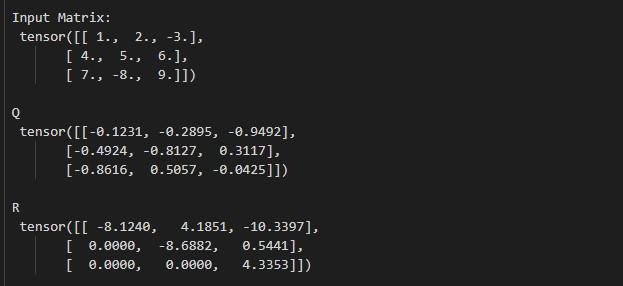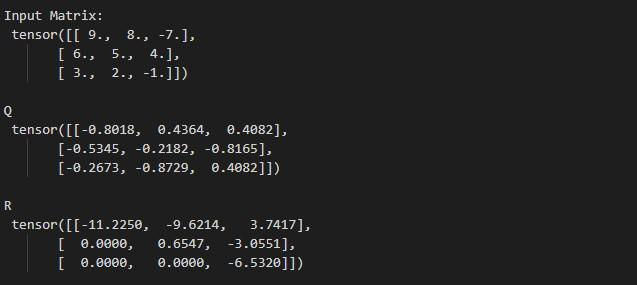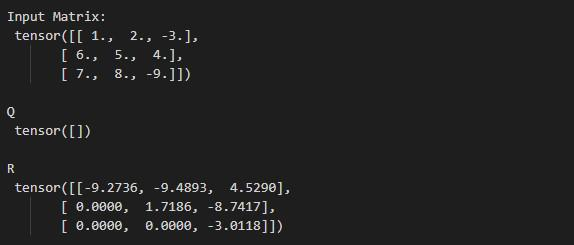Open in App
Not now

# How to compute QR decomposition of a matrix in Pytorch?

• Last Updated : 09 Oct, 2022

In this article, we are going to discuss how to compute the QR decomposition of a matrix in Python using PyTorch.

torch.linalg.qr() method accepts a matrix and a batch of matrices as input. This method also supports the input of float, double, cfloat, and cdouble data types. It will return a named tuple (Q, R). Q is orthogonal in the real case and unitary in the complex case. R is the upper triangular with a real diagonal. The below syntax is used to compute the QR decomposition of a matrix.

Syntax: (Q, R) = torch.linalg.qr(matrix, mode)

Parameters:

• matrix (Tensor): input matrix.
• mode (str, optional): It’s an optional parameter. We have three modes reduced, complete, and r. Default value of this parameter is reduced.

Return: This method return a named tuple (Q, R).

Example 1:

In this example, we will understand how to compute the QR decomposition of a matrix in Pytorch.

## Python3

 `# Import the required libraries``import` `torch`` ` `# create a matrix of size 3x3``matrix ``=` `torch.tensor([[``1.``, ``2.``, ``-``3.``], [``4.``, ``5.``, ``6.``], [``7.``, ``-``8.``, ``9.``]])`` ` `# display input matrix``print``(``"\n Input Matrix: \n"``, matrix)`` ` `# compute the QR decomposition of input matrix``Q, R ``=` `torch.linalg.qr(matrix)`` ` `# display result``print``(``"\n Q \n"``, Q)``print``(``"\n R \n"``, R)`

Output:QR Decomposition of Square Matrix

Example 2:

In this example, we compute the QR decomposition of a matrix. and set the mode to complete.

## Python3

 `# Import the required libraries``import` `torch`` ` `# create a matrix of size 3x3``matrix ``=` `torch.tensor([[``9.``, ``8.``, ``-``7.``], [``6.``, ``5.``, ``4.``], [``3.``, ``2.``, ``-``1.``]])`` ` `# display input matrix``print``(``"\n Input Matrix: \n"``, matrix)`` ` `# compute the QR decomposition of input matrix``Q, R ``=` `torch.linalg.qr(matrix, mode``=``'r'``)`` ` `# display result``print``(``"\n Q \n"``, Q)``print``(``"\n R \n"``, R)`

Output:QR Decomposition of Square Matrix

Example 3:

In this example, we compute the QR decomposition of a matrix. and set the mode to R.

## Python3

 `# Import the required libraries``import` `torch`` ` `# create a matrix of size 3x3``matrix ``=` `torch.tensor([[``1.``, ``2.``, ``-``3.``], [``6.``, ``5.``, ``4.``], [``7.``, ``8.``, ``-``9.``]])`` ` `# display input matrix``print``(``"\n Input Matrix: \n "``, matrix)`` ` `# compute the QR decomposition of input matrix``Q, R ``=` `torch.linalg.qr(matrix, mode``=``'r'``)`` ` `# display result``print``(``"\n Q \n "``, Q)``print``(``"\n R \n "``, R)`

Output:QR Decomposition of Square Matrix

My Personal Notes arrow_drop_up